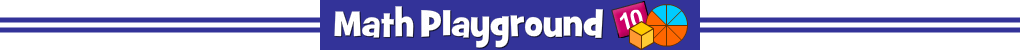Advertisement
Math Millionaire (Flash)
Math at the Mall 2 - Learning Connections
Essential Skills
Real World Math - find discounts, tip,s and sale prices
Mental Math - calculate the percent of a number

Common Core Connection for Grades 5+
Express fractions as percentages of a whole.
Understand that percentages are another way to express fractions.
Understand decimal notation for fractions.
Understand the place value system.
More Math Games to Play